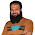### CHAPTER 6 : Fluid Dynamics

(1)               Coefficient of viscosity of honey is less than.

(a)     milk
(b)    water
(c)     tarcoal
(d)    water

(2)               The dimensions of coefficient of viscosity are.
(a)     ML-1T-1
(b)    M2L1T1
(c)     ML1T-1
(d)    M2L-1T-1

(3)               Terminal velocity is.
(a)     uniform
(b)    maximum
(c)     uniform and maximum
(d)    neither uniform nor maximum

(4)               When body moves with terminal velocity the acceleration in the body become.
(a)     zero
(b)    maximum
(c)     variable
(d)    infinite

(5)               Terminal velocity is given by equation.
(a)     Vt = gr2r
(b)    Vt = gr2r/9η
(c)     Vt = gr2r/9η
(d)    Vt = 9gr2r/2η

(6)               Terminal velocity of the body is directly proportional to the.
(a)  radius of the body
(a)     diameter of the body
(b)    size of the body
(c)     square of the diameter of the body

(7)               The flow of ideal fluid is always.
(a)     turbulent
(b)    streamline
(c)     irregular
(d)    straight line

(8)               Drag force is given by.
(a)     Newton’s law
(b)    Pascal’s law
(c)     Gauss’s law
(d)    Stoke’s law

(9)               When fluid is incompressible then.
(a)     velocity of the fluid is constant
(b)    flow of the fluid is constant
(c)     density of the fluid is constant
(d)    volume of the fluid is constant

(10)           Irregular flow of fluid is called.
(a)     streamline
(b)    turbulent
(c)     uniform
(d)    laminar

(11)      According to equation of continuity,   A1V1  =  A2V2          =   constant.
The constant is equal to.
(a)     flow rate
(b)    volume of fluid
(c)     mass of fluid
(d)    density of fluid

(12)      Equation of continuity is obtained by apply in law of conservation
of.
(e)     mass
(f)     energy
(g)     momentum
(h)    all

(13)           Velocity of fluid increases where the pressure is.
(a)     low
(b)    high
(c)     constant
(d)    changes continuously
(14)           Speed of efflux can be determined by applying.
(a)     Bernoull’s theorem
(b)    Torricelli’s theorem
(c)     Venture relation
(d)    All

(15)           Blood vessels are.
(a)     rigid
(b)    not rigid
(c)     of glass
(d)    of rubber

(16)           Concentration of red cells in blood is about.
(a)     25%
(b)    40%
(c)     50%
(d)    75%

(17)           A man standing near a fast moving train may fall.
(a)     on the train
(b)    away from the train
(c)     towards the train
(d)    on himself

(18)           For which position, maximum blood pressure in the body have the smallest value ?
(a)     standing straight
(b)    sitting on chair
(c)     sitting on ground
(d)    lying horizontally

(19)           Two fog droplets have radius 2:3, their terminal velocities are .
(a)     4:6
(b)    4:9
(c)     2:9
(d)    4:3

(20)           Burnoulli’s equation is obtained by applying law of conservation
of .
(a)     mass
(b)    energy
(c)     momentum
(d)    fluid

(21)           Venturi meter is used to measure.
(a)     fluid pressure
(b)    fluid density
(c)     fluid speed
(d)    none

(22)           In cricket when a bowler produce reverse swing, the ball will move
towards.
(a)     Shinning side of the ball
(b)    Rough side
(c)     Seam of the ball
(d)    Goes straight

(23)           Stokes law is applicable if body has __________ shape.
(a)     rough
(b)    square
(c)     circular
(d)    spherical

(24)           One torr is equal to.
(a)     1.333 Nm-2
(b)    .1333 Nm-2
(c)     13.33 Nm-2
(d)    133.3 Nm-2

(25)           Systolic pressure is called.
(a)     low blood pressure
(b)    high blood pressure
(c)     normal blood pressure
(d)    abnormal blood pressure

(26)           Instrument used to measure blood pressure is called.
(a)     Venturimeter
(b)    Blood pressure
(c)     Sphygmomanometer
(d)    Sonometer

(27)           A chimney work best if air exposed to the chimney is,
(a)     Stationary
(b)    Moving
(c)     Moving slowly
(d)    Moving fast

(28)           Which  one is venture relation?
(a)     P1 – P2  =  1 / 2 ρV22
(b)    V2 = 2g (h1-h2)
(c)     P + 1 / 2ρV2 + ρgh = Constant
(d)    A1V1 = A2V2 = Constant

(29)           The effect of the decrease in pressure with the increase of the speed of fluid in a horizontal pipe is known as.
(a)     Bernoulli’s effect
(b)    Torricelli’s effect
(c)     Venture effect
(d)    Stokes effect

(30)           Ideal fluid is.
(a)     non-viscous
(b)    incompressible
(c)     steady flow
(d)    possess all properties

(31)           When weight of an object falling freely becomes equal to the drag force, then the body will move with
(a)   increasing speed
(b)   decreasing speed
(c)   constant speed
(d)   none of them

(32)        The body will move with terminal velocity when it acquires
(a)   minimum speed
(b)   zero speed
(c)   maximum speed
(d)   none of them

(33)        When the body reaches its terminal velocity, the acceleration of the body becomes
(a)     maximum speed
(b)     minimum speed
(c)   zero
(d)   constant quantity

(34)        A water hose with an internal diameter of 20 mm at the outlet discharges 30 kg of water in 60 s. what is water speed at the outlet if density of water is 1000 Kg/m3 during its steady flow.
(a)   1.3 m/s         (b)   1.6m/s
(c)   1.9 m/s         (d)   2.2 m/s

(35)        The direction of the streamlines is the same as the direction of the
(a)   force            (b)   torque
(c)   velocity        (d)   weight

(36)        When the different streamlines cannot cross each other, then this condition is known as
(a)   continuity condition
(b)   turbulent flow condition
(c)   steady flow condition
(d)   none of them

(37)        When each particle of the fluid moves along a smooth path, this path is known as
(a)     straight path
(b)   smooth path
(c)   haphazard path
(d)   streamline

(38)        During the steady flow, different streamlines
(a)   cannot across each other
(b)   can across each other
(c)   either of them
(d)   neither of them

(39)        If every particle of the flow that passes a particular point, moves along the same path as followed by particles which passed the point earlier, then this flow is said to be
(a)   turbulent      (b)   streamline
(d)   abrupt          (d)   none of them

(40)        When a fluid is in motion, its flow can be considered as
(a)      turbulent
(b)   streamline
(c)   either of them
(d)   neither of them

### Comments

1.1.